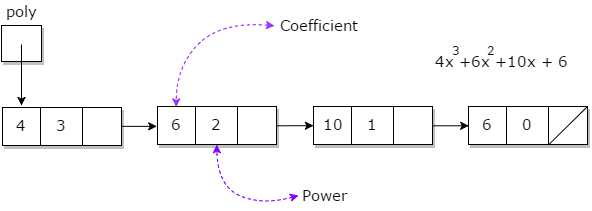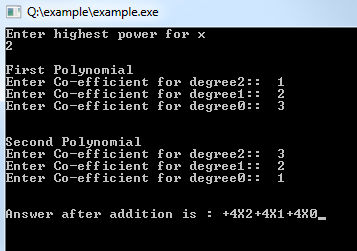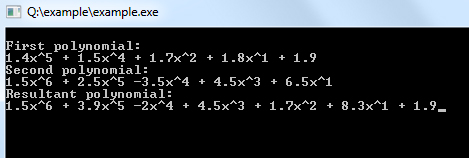# Polynomials Using Linked List and Arrays

Polynomials and Sparse Matrix are two important applications of arrays and linked lists. A polynomial is composed of different terms where each of them holds a coefficient and an exponent. This tutorial chapter includes the representation of polynomials using linked lists and arrays.

## What is Polynomial?

A polynomial p(x) is the expression in variable x which is in the form (axn + bxn-1 + …. + jx+ k), where a, b, c …., k fall in the category of real numbers and 'n' is non negative integer, which is called the degree of polynomial.

An essential characteristic of the polynomial is that each term in the polynomial expression consists of two parts:

• one is the coefficient
• other is the exponent

Example:

10x2 + 26x, here 10 and 26 are coefficients and 2, 1 is its exponential value.

Points to keep in Mind while working with Polynomials:

• The sign of each coefficient and exponent is stored within the coefficient and the exponent itself
• Additional terms having equal exponent is possible one
• The storage allocation for each term in the polynomial must be done in ascending and descending order of their exponent## Representation of Polynomial

Polynomial can be represented in the various ways. These are:

• By the use of arrays
• By the use of Linked List

### Representation of Polynomials Using Arrays

There may arise some situation where you need to evaluate many polynomial expressions and perform basic arithmetic operations like addition and subtraction with those numbers. For this, you will have to get a way to represent those polynomials. The simple way is to represent a polynomial with degree 'n' and store the coefficient of n+1 terms of the polynomial in the array. So every array element will consist of two values:

• Coefficient and
• Exponent

A polynomial can be represented using the C++ code:

``````#include <iostream>
#include <iomanip.h>
using namespace std;

struct poly {
int coeff;
int pow_val;
poly* next;
};

poly *poly1, *poly2, *poly3;

public:
add() { poly1 = poly2 = poly3 = NULL; }
void display();
};

{
int i, p;
poly *newl = NULL, *end = NULL;
cout << "Enter highest power for x\n"; cin >> p;
cout << "\nFirst Polynomial\n"; for (i = p; i >= 0; i--) {
newl = new poly;
newl->pow_val = p;
cout << "Enter Co-efficient for degree" << i << ":: "; cin >> newl->coeff;
newl->next = NULL;
if (poly1 == NULL)
poly1 = newl;
else
end->next = newl;
end = newl;
}

cout << "\n\nSecond Polynomial\n"; end = NULL; for (i = p; i >= 0; i--) {
newl = new poly;
newl->pow_val = p;
cout << "Enter Co-efficient for degree" << i << ":: "; cin >> newl->coeff;
newl->next = NULL;
if (poly2 == NULL)
poly2 = newl;
else
end->next = newl;
end = newl;
}

poly *p1 = poly1, *p2 = poly2;
end = NULL;
while (p1 != NULL && p2 != NULL) {
if (p1->pow_val == p2->pow_val) {
newl = new poly;
newl->pow_val = p--;
newl->coeff = p1->coeff + p2->coeff;
newl->next = NULL;
if (poly3 == NULL)
poly3 = newl;
else
end->next = newl;
end = newl;
}
p1 = p1->next;
p2 = p2->next;
}
}

{
poly* t = poly3;
while (t != NULL) {
cout.setf(ios::showpos);
cout << t->coeff;
cout.unsetf(ios::showpos);
cout << "X" << t->pow_val;
t = t->next;
}
}
int main()
{
obj.display();
}
``````

Output:### Representation of Polynomial Using Linked Lists

A polynomial can be thought of as an ordered list of non zero terms. Each non zero term is a two-tuple which holds two pieces of information:

• The exponent part
• The coefficient part

### Program for the addition of Polynomials

``````#include <iostream>
using namespace std;

class polyll {
private:
struct polynode {
float coeff;
int exp;
} * p;

public:
polyll();
void poly_append(float c, int e);
void display_poly();
~polyll();
};
polyll::polyll()
{
p = NULL;
}
void polyll::poly_append(float c, int e)
{
polynode* temp = p;
if (temp == NULL) {
temp = new polynode;
p = temp;
}
else {
}
temp->coeff = c;
temp->exp = e;
}
void polyll::display_poly()
{
polynode* temp = p;
int f = 0;

cout << endl; while (temp != NULL) { if (f != 0) { if (temp->coeff > 0)
cout << " + ";
else
cout << " "; } if (temp->exp != 0)
cout << temp->coeff << "x^" << temp->exp;
else
cout << temp->coeff;
f = 1;
}
}
{
polynode* z;
if (l1.p == NULL && l2.p == NULL)
return;
polynode *temp1, *temp2;
temp1 = l1.p;
temp2 = l2.p;
while (temp1 != NULL && temp2 != NULL) {
if (p == NULL) {
p = new polynode;
z = p;
}
else {
}
if (temp1->exp < temp2->exp) {
z->coeff = temp2->coeff;
z->exp = temp2->exp;
}
else {
if (temp1->exp > temp2->exp) {
z->coeff = temp1->coeff;
z->exp = temp1->exp;
}
else {
if (temp1->exp == temp2->exp) {
z->coeff = temp1->coeff + temp2->coeff;
z->exp = temp1->exp;
}
}
}
}
while (temp1 != NULL) {
if (p == NULL) {
p = new polynode;
z = p;
}
else {
}
z->coeff = temp1->coeff;
z->exp = temp1->exp;
}
while (temp2 != NULL) {
if (p == NULL) {
p = new polynode;
z = p;
}
else {
}
z->coeff = temp2->coeff;
z->exp = temp2->exp;
}
}
polyll::~polyll()
{
polynode* q;
while (p != NULL) {
delete p;
p = q;
}
}
int main()
{
polyll p1;
p1.poly_append(1.4, 5);
p1.poly_append(1.5, 4);
p1.poly_append(1.7, 2);
p1.poly_append(1.8, 1);
p1.poly_append(1.9, 0);
cout << "\nFirst polynomial:";
p1.display_poly();
polyll p2;
p2.poly_append(1.5, 6);
p2.poly_append(2.5, 5);
p2.poly_append(-3.5, 4);
p2.poly_append(4.5, 3);
p2.poly_append(6.5, 1);
cout << "\nSecond polynomial:";
p2.display_poly();
polyll p3;